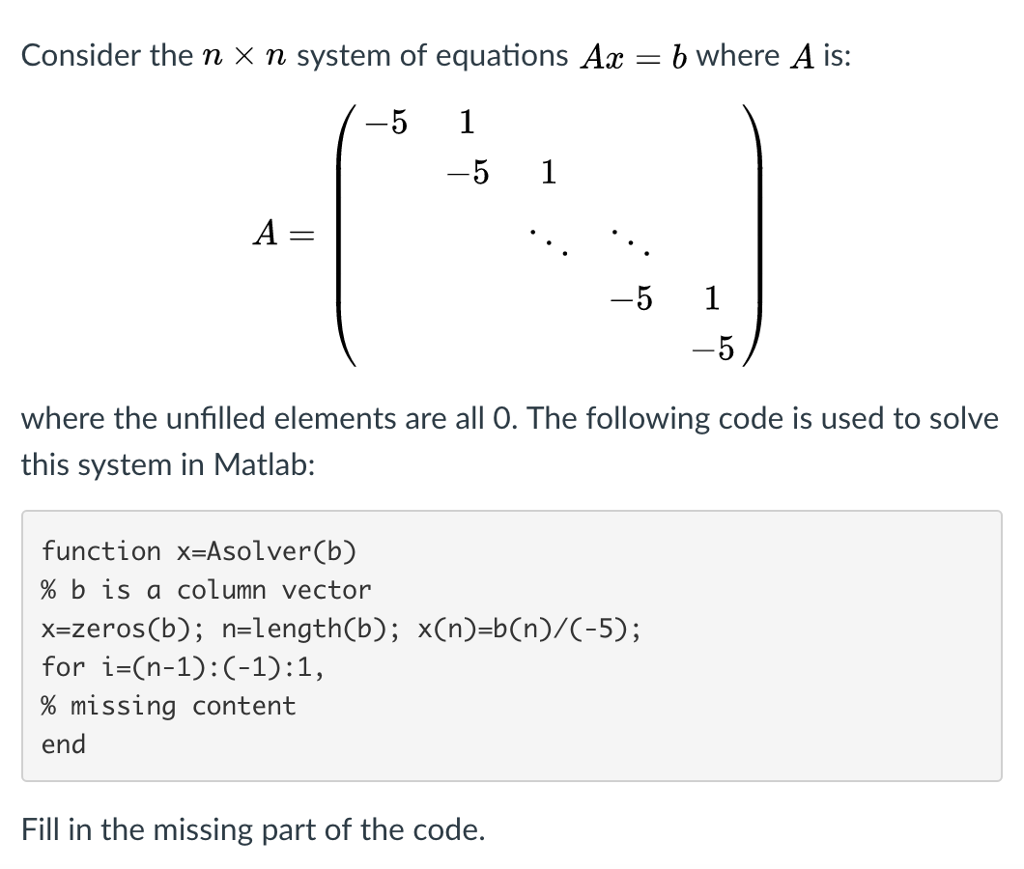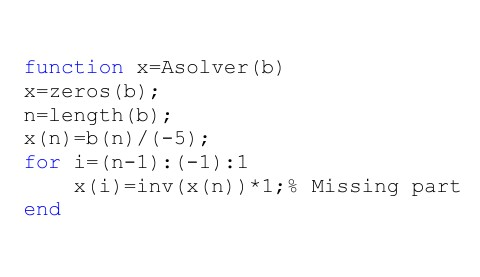# Question & Answer: Consider the n times n system of equations Ax = b where A is: A = (-5 1 -5 1 -5 1 -5) where the unfilled elements are all 0…..Consider the n times n system of equations Ax = b where A is: A = (-5 1 -5 1 -5 1 -5) where the unfilled elements are all 0. The following code is used to solve this system in Matlab: function x=Asolver(b) % b is a column vector x=zeros(b): n=length(b): x(n)=b(n)/(-5): for i=(n-1): (-1): 1, % missing content end Fill in the missing part of the code.